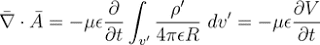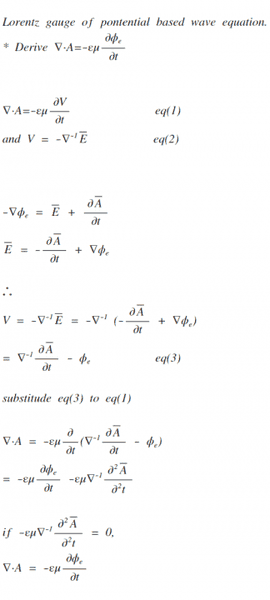# [electromagnetics] Lorentz Gauge

kidsasd987I am trying to derive the potential based Lorentz gauge, but I am not sure if I am on the right track.
Why the second partial derivative of vector potential must be 0?

Please correct me where I got this wrong.

Last edited:

Gold Member
2022 Award
Let's start from scratch, i.e., Maxwell's equations and use convenient Heaviside Lorentz units. The Maxwell equations divide into two groups, the homogeneous ones
$$\frac{1}{c} \partial_t \vec{B}+\vec{\nabla} \times \vec{E}=0, \quad \vec{\nabla} \cdot \vec{B}=0$$
and the inhomogeneous ones
$$-\frac{1}{c} \partial_t \vec{E} + \vec{\nabla} \times \vec{B}=\frac{1}{c} \vec{j}, \quad \vec{\nabla} \cdot \vec{E}=\rho.$$
The homogeneous Maxwell equations are just constraints on the fields, and it is often more convenient to reduce the degrees of freedom. This is achieved by introducing first a vector potential for ##\vec{B}##. According to Helmholtz's fundamental theorem of vector calculus because the divergence of ##\vec{B}## vanishes, it must be a pure curl of a vector field,
$$\vec{B}=\vec{\nabla} \times \vec{A}.$$
this you plug into the first homogeneous equation (the Fraday law of induction) leading to
$$\vec{\nabla} \times \left (\frac{1}{c} \partial_t \vec{A} + \vec{E} \right)=0.$$
Again using Helmholtz's fundamental theorem, you conclude that the expression in the parenthesis must be the gradient of a scalar field,
$$\frac{1}{c} \partial_t \vec{A} + \vec{E}=-\vec{\nabla} \Phi.$$
The choice of the signs is just convention. Now you have solved the homogeneous equations by introducing the potentials via
$$\vec{B}=\vec{\nabla} \times \vec{A}, \quad \vec{E}=-\frac{1}{c} \partial_t \vec{A} -\vec{\nabla} \Phi.$$
Now for a given field configuration ##\vec{E}## and ##\vec{B}## these potentials are not unique. You can add a gradient to the vector potential without changing the magnetic field, i.e.,
$$\vec{A}'=\vec{A}-\nabla \chi$$
leads to the same magnetic field, because ##\vec{\nabla} \times (\vec{\nabla} \chi)=0## for any scalar field ##\chi##. To also make the electric field right, you have to introduce a new scalar potential too, i.e., you want
$$\vec{E}=-\frac{1}{c} \partial_t \vec{A}'-\vec{\nabla} \Phi'=-\frac{1}{c} \partial_t \vec{A} + \vec{\nabla} \left (\frac{1}{c} \partial_t \chi-\Phi' \right),$$
i.e., you can choose ##\Phi'## such that
$$\frac{1}{c} \partial_t \chi - \Phi'=-\Phi \; \Rightarrow \; \Phi'=\Phi+\frac{1}{c} \partial_t \chi.$$
Thus with any scalar field ##\chi## the potentials
$$\vec{A}'=\vec{A}-\vec{\nabla} \chi, \quad \Phi'=\Phi+\frac{1}{c} \partial_t \chi$$
describe the same physical situation as the original potentials. This is called gauge invariance of electrodynamics.

This means you have some freedom to choose your potentials conveniently. To see what's most convenient we use the inhomogeneous Maxwell equations. Plugging in the potentials gives
$$\vec{\nabla} \times \vec{B}-\frac{1}{c} \partial_t \vec{E} = \vec{\nabla} \times (\vec{\nabla} \times \vec{A})-\frac{1}{c} \partial_t \left (-\frac{1}{c} \partial_t \vec{A}- \vec{\nabla} \Phi \right )=\frac{1}{c} \vec{j}.$$
The first expression can be simplified (in Cartesian coordinates!) to
$$\vec{\nabla} \times (\vec{\nabla} \times \vec{A})=\vec{\nabla}(\vec{\nabla} \cdot \vec{A})-\Delta \vec{A},$$
i.e., the Ampere-Maxwell Law becomes
$$\Box \vec{A} + \vec{\nabla} \left (\frac{1}{c} \partial_t \Phi + \vec{\nabla} \cdot \vec{A} \right)=\frac{1}{c} \vec{j},$$
where
$$\Box=\frac{1}{c^2} \partial_t^2 -\Delta$$
is the D'Alembert operator. Obviously the above equations become most convenient, when we choose the potentials such that the parentheses vanish, because then the equation of motion for the vector potential separates into three wave equations for each component separately. We are free to so because of the gauge invariance, i.e., we can impose the constraint
$$\frac{1}{c} \partial_t \Phi+\vec{\nabla} \cdot \vec{A}=0,$$
which is a socalled gauge-fixing constraint. It's known as the Lorenz gauge (in the older literature somwhat injustly called the Lorentz gauge, mixing up the historical order, because it was the Danish physicist Ludvig Lorenz who found this particular gauge fixing rather than the Dutch physicist Hendrik A. Lorentz).

Now fortunately also the remaining equation becomes simple with the choice of the Lorenz gauge, because
$$-\vec{\nabla} \cdot \left (\frac{1}{c} \partial_t \vec{A}+\vec{\nabla} \Phi \right )=\rho.$$
Now we have because of the Lorenz-gauge condition
$$\frac{1}{c} \partial_t \vec{\nabla} \cdot \vec{A}=-\frac{1}{c^2} \partial_t^2 \Phi,$$
and thus
$$\Box \Phi=\rho.$$
In the Lorenz gauge thus also the scalar potential separates from the vector potential and fulfills a simple inhomogeneous wave equation, which in the usual physical situations, where you like to calculate the emission of electromagnetic waves from given charge-current distributions, is solved by the retarded Green's function, leading to the retarded potentials.

•dextercioby
Gold Member
2022 Award
I think those who are responsible for giving justice to Lorenz are Jackson and Okun with this very interesting article in RMP:

Jackson, J. D., Okun, L. B.: Historical roots of gauge invariance, Rev. Mod. Phys. 73(3), 663–680, 2001

or as preprint

•dextercioby
kidsasd987
Let's start from scratch, i.e., Maxwell's equations and use convenient Heaviside Lorentz units. The Maxwell equations divide into two groups, the homogeneous ones
$$\frac{1}{c} \partial_t \vec{B}+\vec{\nabla} \times \vec{E}=0, \quad \vec{\nabla} \cdot \vec{B}=0$$
and the inhomogeneous ones
$$-\frac{1}{c} \partial_t \vec{E} + \vec{\nabla} \times \vec{B}=\frac{1}{c} \vec{j}, \quad \vec{\nabla} \cdot \vec{E}=\rho.$$
The homogeneous Maxwell equations are just constraints on the fields, and it is often more convenient to reduce the degrees of freedom. This is achieved by introducing first a vector potential for ##\vec{B}##. According to Helmholtz's fundamental theorem of vector calculus because the divergence of ##\vec{B}## vanishes, it must be a pure curl of a vector field,
$$\vec{B}=\vec{\nabla} \times \vec{A}.$$
this you plug into the first homogeneous equation (the Fraday law of induction) leading to
$$\vec{\nabla} \times \left (\frac{1}{c} \partial_t \vec{A} + \vec{E} \right)=0.$$
Again using Helmholtz's fundamental theorem, you conclude that the expression in the parenthesis must be the gradient of a scalar field,
$$\frac{1}{c} \partial_t \vec{A} + \vec{E}=-\vec{\nabla} \Phi.$$
The choice of the signs is just convention. Now you have solved the homogeneous equations by introducing the potentials via
$$\vec{B}=\vec{\nabla} \times \vec{A}, \quad \vec{E}=-\frac{1}{c} \partial_t \vec{A} -\vec{\nabla} \Phi.$$
Now for a given field configuration ##\vec{E}## and ##\vec{B}## these potentials are not unique. You can add a gradient to the vector potential without changing the magnetic field, i.e.,
$$\vec{A}'=\vec{A}-\nabla \chi$$
leads to the same magnetic field, because ##\vec{\nabla} \times (\vec{\nabla} \chi)=0## for any scalar field ##\chi##. To also make the electric field right, you have to introduce a new scalar potential too, i.e., you want
$$\vec{E}=-\frac{1}{c} \partial_t \vec{A}'-\vec{\nabla} \Phi'=-\frac{1}{c} \partial_t \vec{A} + \vec{\nabla} \left (\frac{1}{c} \partial_t \chi-\Phi' \right),$$
i.e., you can choose ##\Phi'## such that
$$\frac{1}{c} \partial_t \chi - \Phi'=-\Phi \; \Rightarrow \; \Phi'=\Phi+\frac{1}{c} \partial_t \chi.$$
Thus with any scalar field ##\chi## the potentials
$$\vec{A}'=\vec{A}-\vec{\nabla} \chi, \quad \Phi'=\Phi+\frac{1}{c} \partial_t \chi$$
describe the same physical situation as the original potentials. This is called gauge invariance of electrodynamics.

This means you have some freedom to choose your potentials conveniently. To see what's most convenient we use the inhomogeneous Maxwell equations. Plugging in the potentials gives
$$\vec{\nabla} \times \vec{B}-\frac{1}{c} \partial_t \vec{E} = \vec{\nabla} \times (\vec{\nabla} \times \vec{A})-\frac{1}{c} \partial_t \left (-\frac{1}{c} \partial_t \vec{A}- \vec{\nabla} \Phi \right )=\frac{1}{c} \vec{j}.$$
The first expression can be simplified (in Cartesian coordinates!) to
$$\vec{\nabla} \times (\vec{\nabla} \times \vec{A})=\vec{\nabla}(\vec{\nabla} \cdot \vec{A})-\Delta \vec{A},$$
i.e., the Ampere-Maxwell Law becomes
$$\Box \vec{A} + \vec{\nabla} \left (\frac{1}{c} \partial_t \Phi + \vec{\nabla} \cdot \vec{A} \right)=\frac{1}{c} \vec{j},$$
where
$$\Box=\frac{1}{c^2} \partial_t^2 -\Delta$$
is the D'Alembert operator. Obviously the above equations become most convenient, when we choose the potentials such that the parentheses vanish, because then the equation of motion for the vector potential separates into three wave equations for each component separately. We are free to so because of the gauge invariance, i.e., we can impose the constraint
$$\frac{1}{c} \partial_t \Phi+\vec{\nabla} \cdot \vec{A}=0,$$
which is a socalled gauge-fixing constraint. It's known as the Lorenz gauge (in the older literature somwhat injustly called the Lorentz gauge, mixing up the historical order, because it was the Danish physicist Ludvig Lorenz who found this particular gauge fixing rather than the Dutch physicist Hendrik A. Lorentz).

Now fortunately also the remaining equation becomes simple with the choice of the Lorenz gauge, because
$$-\vec{\nabla} \cdot \left (\frac{1}{c} \partial_t \vec{A}+\vec{\nabla} \Phi \right )=\rho.$$
Now we have because of the Lorenz-gauge condition
$$\frac{1}{c} \partial_t \vec{\nabla} \cdot \vec{A}=-\frac{1}{c^2} \partial_t^2 \Phi,$$
and thus
$$\Box \Phi=\rho.$$
In the Lorenz gauge thus also the scalar potential separates from the vector potential and fulfills a simple inhomogeneous wave equation, which in the usual physical situations, where you like to calculate the emission of electromagnetic waves from given charge-current distributions, is solved by the retarded Green's function, leading to the retarded potentials.

I appreciate you for your detailed comment.
I am an engineering student so, it took me a while to catch up heavyside Lorentz Unit, but wow. This is indeed beautiful.

Ah, and stupid me.. I should've not used inverse del operator..

I have one more question.

1.

Now for a given field configuration E&#x2192;" role="presentation" style="display: inline-block; line-height: 0; font-size: 14.69px; word-wrap: normal; white-space: nowrap; float: none; direction: ltr; max-width: none; max-height: none; min-width: 0px; min-height: 0px; border: 0px; margin: 0px; padding: 1px 0px; font-family: 'PT Sans', san-serif; position: relative; background-color: rgb(255, 255, 255);">⃗EE→ and B&#x2192;" role="presentation" style="display: inline-block; line-height: 0; font-size: 14.69px; word-wrap: normal; white-space: nowrap; float: none; direction: ltr; max-width: none; max-height: none; min-width: 0px; min-height: 0px; border: 0px; margin: 0px; padding: 1px 0px; font-family: 'PT Sans', san-serif; position: relative; background-color: rgb(255, 255, 255);">⃗BB→ these potentials are not unique. You can add a gradient to the vector potential without changing the magnetic field, i.e.,

Isn't B uniquely determined as we know that divergence of B is 0 and curl of A is a non-zero vector field B. Did you mean to say vector potential A here? or because A is inderminate so B is also not uniquely determined?

Thank you!

Last edited: